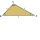# Triangles

Hanka cut the 20 cm long straws into three pieces each piece had a length in cm. Then, with these three pieces, she tried to make a triangle.

a) What circuit has each of the triangles?
b) How long can the longest side measure?
c) How many different triangles could be made?

Result

o =  20 cm
a =  9 cm
n =  8

#### Solution:Leave us a comment of this math problem and its solution (i.e. if it is still somewhat unclear...):Be the first to comment!#### Following knowledge from mathematics are needed to solve this word math problem:

Do you want to convert length units? See also our trigonometric triangle calculator.

## Next similar math problems:

1. TrianglesFive sticks with a length of 2,3,4,5,6 cm. How many ways can you choose three sticks to form three sides of a triangle?
2. TrianglesIvo wants to draw all the triangles whose two sides of which have a length of 4 cm and 9 cm and the length of the third side is expressed in whole centimeters. How many triangles does he have?
3. TriangleProve whether you can construct a triangle ABC, if a=9 cm, b=6 cm, c=10 cm.